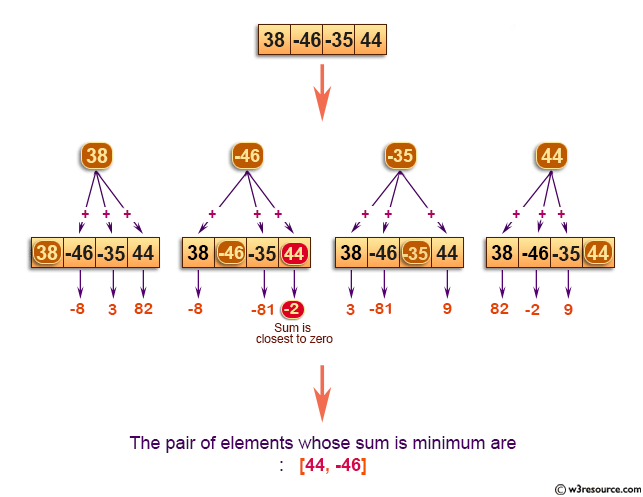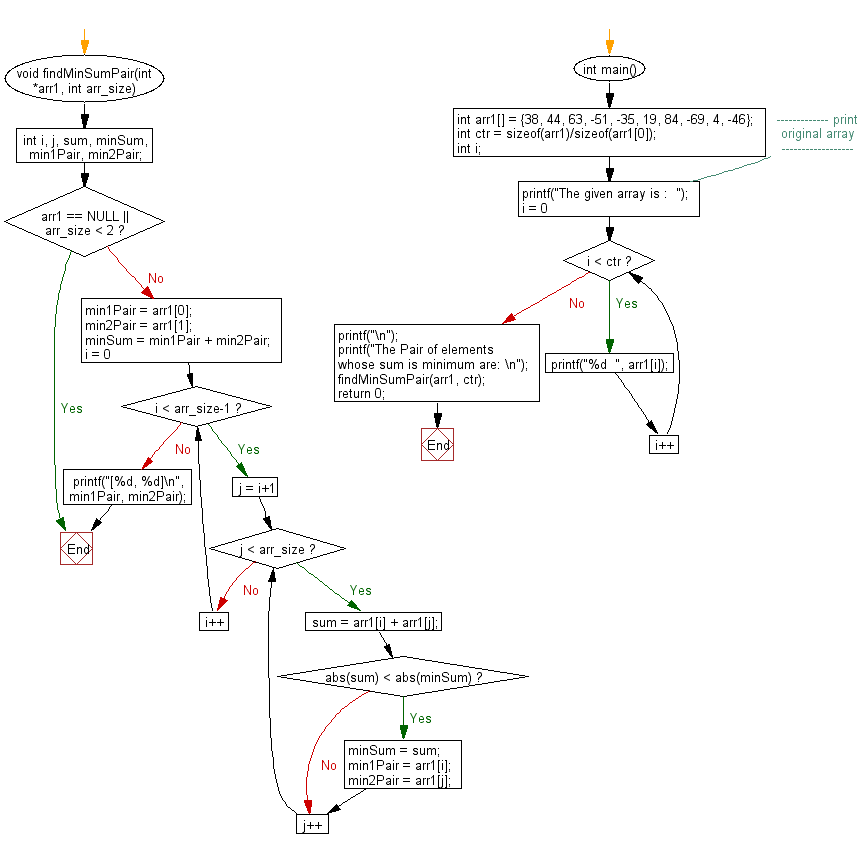﻿ C exercises: Find two elements whose sum is closest to zero - w3resource# C Exercises: Find two elements whose sum is closest to zero

## C Array: Exercise-45 with Solution

Write a program in C to find two elements whose sum is closest to zero.

Pictorial Presentation:Sample Solution:

C Code:

``````#include <stdio.h>
#include <math.h>
#include <stdlib.h>

void findMinSumPair(int *arr1, int arr_size)
{
int i, j, sum, minSum, min1Pair, min2Pair;

if(arr1 == NULL || arr_size < 2)
return;
min1Pair = arr1;
min2Pair = arr1;
minSum = min1Pair + min2Pair;

for(i = 0; i < arr_size-1; i++)
{
for(j = i+1; j < arr_size; j++)
{
sum = arr1[i] + arr1[j];
if(abs(sum) < abs(minSum))
{
minSum = sum;
min1Pair = arr1[i];
min2Pair = arr1[j];
}
}
}
printf("[%d, %d]\n", min1Pair, min2Pair);
}

int main()
{
int arr1[] = {38, 44, 63, -51, -35, 19, 84, -69, 4, -46};
int ctr = sizeof(arr1)/sizeof(arr1);
int i;
//------------- print original array ------------------
printf("The given array is :  ");
for(i = 0; i < ctr; i++)
{
printf("%d  ", arr1[i]);
}
printf("\n");
//------------------------------------------------------
printf("The Pair of elements whose sum is minimum are: \n");
findMinSumPair(arr1, ctr);
return 0;
}
```
```

Sample Output:

```The given array is :  38  44  63  -51  -35  19  84  -69  4  -46
The Pair of elements whose sum is minimum are:
[44, -46]
```

Flowchart:C Programming Code Editor:

Improve this sample solution and post your code through Disqus.

What is the difficulty level of this exercise?

﻿